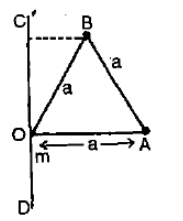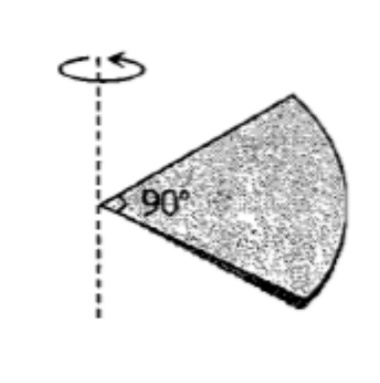#17 | Set 1: Previous Year Problems
(Physics) > Systems of Particles and Rotational Motion
Unable to watch the video, please try another server

#### My Notes

Create your notes while watching video by clicking on icon in video player.
Related Practice Questions :

Three-point masses 'm' each, are placed at the vertices of an equilateral triangle of side a. Moment of inertia of the system about axis COD is-1. $2m{a}^{2}$

2. $\frac{2}{3}m{a}^{2}$

3. $\frac{5}{4}m{a}^{2}$

4. $\frac{7}{4}m{a}^{2}$

NEET 2021 - Achiever Batch - Aryan Raj Singh

Difficulty Level:

One solid sphere A and another hollow sphere B are of same mass and same outer radii. Their moment of inertia about their diameters are respectively IA and IB such that

1. ${\mathrm{I}}_{\mathrm{A}}={\mathrm{I}}_{\mathrm{B}}$

2. ${\mathrm{I}}_{\mathrm{A}}>{\mathrm{I}}_{\mathrm{B}}$

3. ${\mathrm{I}}_{\mathrm{A}}<{\mathrm{I}}_{\mathrm{B}}$

4. $\frac{{\mathrm{I}}_{\mathrm{A}}}{{\mathrm{I}}_{\mathrm{B}}}=\frac{{d}_{A}}{{d}_{B}}$

NEET 2021 - Achiever Batch - Aryan Raj Singh

Difficulty Level:

A particle of mass 1 kg is kept at (1m, 1m, 1m). The moment of inertia of this particle about z-axis would be

1.

2.

3.

4.  None of these

NEET 2021 - Achiever Batch - Aryan Raj Singh

Difficulty Level:

One quarter sector is cut from a uniform circular disc of radius R.  This sector has mass M.  It is made to rotate about a line perpendicular to its plane and passing through the centre of the original disc.  Its moment of inertia about the axis of rotation is [IIT-JEE (Screening) 2001](1) $\frac{1}{2}M{R}^{2}$

(2) $\frac{1}{4}M{R}^{2}$

(3) $\frac{1}{8}M{R}^{2}$

(4) $\sqrt{2}M{R}^{2}$

NEET 2021 - Achiever Batch - Aryan Raj Singh

Difficulty Level:

The radius of gyration of a uniform rod of length L about an axis passing through its centre of mass is

1. $\frac{L}{2\sqrt{3}}$

2. $\frac{{L}^{2}}{12}$

3. $\frac{L}{\sqrt{3}}$

4. $\frac{L}{\sqrt{2}}$